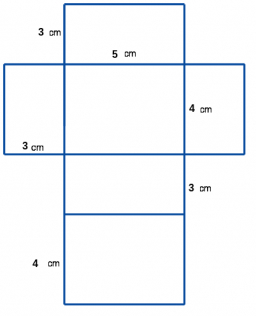# Centimeters 72534

Calculate the edge c and the surface S of a block if its volume is equal to 42 cubic centimeters, a = 6cm, b = 3.5cm, c =?

c =  2 cm
S =  80 cm2

### Step-by-step explanation:Did you find an error or inaccuracy? Feel free to write us. Thank you!

Tips for related online calculators
Need help calculating sum, simplifying, or multiplying fractions? Try our fraction calculator.